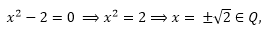Click to Chat

1800-1023-196

+91-120-4616500

CART 0

• 0

MY CART (5)

Use Coupon: CART20 and get 20% off on all online Study Material

ITEM
DETAILS
MRP
DISCOUNT
FINAL PRICE
Total Price: Rs.

There are no items in this cart.
Continue Shopping• Complete JEE Main/Advanced Course and Test Series
• OFFERED PRICE: Rs. 15,900
• View Details

```Chapter 1: Sets – Exercise 1.3

Sets – Exercise 1.3 – Q.1

(i)  This set is non-empty as 10 is an even natural number divisible by 5.

(ii)  As 2 belongs to this set, so it is non-empty.

(iii)the set of rational numbers So, this set is empty.

(iv) This set is empty as there is no natural num bar x such that x < 8 and simultaneously x > 12.

(v) This set is empty as any two parallel lines never intersect each other.

Sets – Exercise 1.3 – Q.2

(i) Infinite, since with a common centre infinitely many circles can be drawn in a plane.

(ii) Finite, as there are only 26 letters of English Alphabet.

(iii) Infinite, ∵ {x ϵ N : x > 5} = {6,7,8,...} which is infinite.

(iv) Finite, ∵ {x ϵ N : x ,200} = {1,2, 3,...199} Which is finite.

(v) Infinite, {x ϵ Z : x < 5} =-{...., -3,-2,-1,0,1,2,3,4,} Which is infinite,

(vi) {x ϵ R : 0 < x < 1) is an infinite set ∵ an interval is an infinite set.

Sets – Exercise 1.3 – Q.3

A = {1,2,3}

B = {x ϵ R : (x - 1)2 = 0}

=  {x ϵ R : x = 1,1}

= {1}

C = {1,2,3} (∵ repetition is not allowed in a set)

D = {x ϵ R : x3 - 6x2 + 11x - 6 = 0}

= {x ϵ R : (x- 1)(x2- 5x + 6) = 0}                  [∵ x = 1 satisfiles the above equation]

= {x ϵ R : (x- 1)(x - 2)(x - 3) = 0}

= {x ϵ R: x = 1, 2, 3}

= {1, 2, 3}

Hence the set A, C and D are equal.

Sets – Exercise 1.3 – Q.4

A = {a, e, p, r}

B = {a, e, p, r} (repetition of 'p' is not allowed)

C = {e, o, p, r}

as A = B ≠ C,  ∴ the sets are not equal

Sets – Exercise 1.3 – Q.5

Two finite sets are said to be equivalent if they have the same number of elements. As A and C have same number of elements, and B and D also have same number of elements.

∴  A is equivalent to C & 8 is equivalent to D.

Sets – Exercise 1.3 – Q.6

(i)

Two sets A and B are said to be equal if every elements of A is an elements of B and vice-versa.

We have, A = {2, 3} and

B = {x : x is a solution of x2 + 5x + 6 = 0

= {x : x2 + 3x + 2x + 6 = 0}

= {x : x (x + 3) +2(x +3 = 0)

= {x: (x + 3)(x +2) = 0}

= {x : x = -2, -3}

= (-2,-3)

Hence A ≠ B.

(ii)

A = {W, O, L, F}

B = {F, O, L, W}                  [∵ repetition is not allowed]

= {W, O, L F}                     [The order in which the elements are written does not matter.]

Hence  A = B

Sets – Exercise 1.3 – Q.7

A = {0, a}

B = {1, 2, 3, 4}

C = {4, 8, 12}

D = {3, 1, 2, 4}

= {1, 2, 3, 4}

E = {1, 0}

F = {8, 4,12}

= {4, 8, 12}

G = {1, 5, 7, 11}

H = {a, b}

The sets B and D are equal.

The sets C and F are equal.

As A, E and H have same number of elements so they are equivalent.

As B, D and G have same number of elements, so they are equivalent

Also C and F have same number of elements, so they are equivalent.

Sets – Exercise 1.3 – Q.8

A = {1, 2}

B = {1, 2}

C = {3, 1}

D = {1, 3}               [∵ the odd natural numbers less than 5 are 1 and 3]

E = {1, 2}                [∵repetition is not allowed]

F = {1, 3}                [∵repetition is not allowed]

∴  A, B and E are equal

Aslo, C, D and F are equal

Sets – Exercise 1.3 – Q.9

The set formed by distinct letters of the word "CATARACT' are (C, A, T ,R).

The set formed by distinct letters of the word "TRACT" are (T, R, A, C}

Hence the two set are equal.
```### Course Features

• 728 Video Lectures
• Revision Notes
• Previous Year Papers
• Mind Map
• Study Planner
• NCERT Solutions
• Discussion Forum
• Test paper with Video Solution